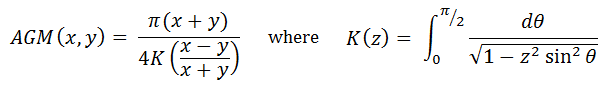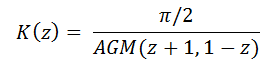# The Arithmetic-Geometric Mean

## And Other Iterated Means

Iterated Mean Calculator
X =           Y =
Select the two means to iterate:

The Arithmetic-Geometric Mean, AGM, is a type of iterative mean, an average one figures by iterating a pair of computations. To calculate the AGM of two numbers x and y, first compute their arithmetic and geometric means, which are

(x+y)/2 and sqrt(xy).

Then compute the arithmetic and geometric means of these two new numbers, and then repeat the process over and over with each new pair of numbers you generate. Eventually, the algorithm stabilizes at a fixed number. This number is the arithmetic-geometric mean of x and y.

Another way to express the arithmetic-geometric mean of x and y is to consider two dependent recursive equations,

An+1 = (An + Bn)/2
Bn+1 = sqrt(AnBn),

where A0 = x and B0 = y. As n goes to infinity, the values of An and Bn converge to a single number, the AGM of x and y. So long as x and y are unequal, the AGM is always greater than the geometric mean and less than the arithmetic mean.

### Elliptic Integral Relation

Although there is no closed form for the arithmetic-geometric mean, it can be expressed in terms of elliptic integrals. If the AGM of x and y is denoted by AGM(x,y) and K(z) is an elliptic integral with parameter z, thenSince elliptic integrals have no closed form solution, the arithmetic geometric mean relation provides an efficient algorithm for computing the value of some types of elliptic integrals numerically.### Other Iterated Means

You can generalize the concept of the arithmetic-geometric mean to create other kinds of iterated averages. See List of Averages for different means and their formulas. With the calculator above, just input the values for x and y, then select the two types of means you wish to apply. The order does not matter, so if you want to compute the AGM, select "Arithmetic" from one dropdown menu and "Geometric" from the other.

#### Geometric-Harmonic

Another example of an iterative average is the Geometric-Harmonic Mean, GHM. If C0 = x and D0 = y, and

Cn+1 = 2CnDn/(Cn + Dn)
Dn+1 = sqrt(CnDn),

then the stable value of the sequence is the GHM of x and y. There are nice relations between AGM(x,y) and GHM(x,y):

AGM(x,y)GHM(x,y) = xy

GHM(x,y) = xy/AGM(x,y) = 1/AGM(x-1, y-1)

#### Arithmetic-Harmonic

If you iterate the arithmetic means and harmonic means, you end up with the geometric mean.

#### Contraharmonic-Harmonic

The contraharmonic mean of x and y is (x2 + y2)/(x+y). If you iterate this with the harmonic mean, you end up with the arithmetic mean.